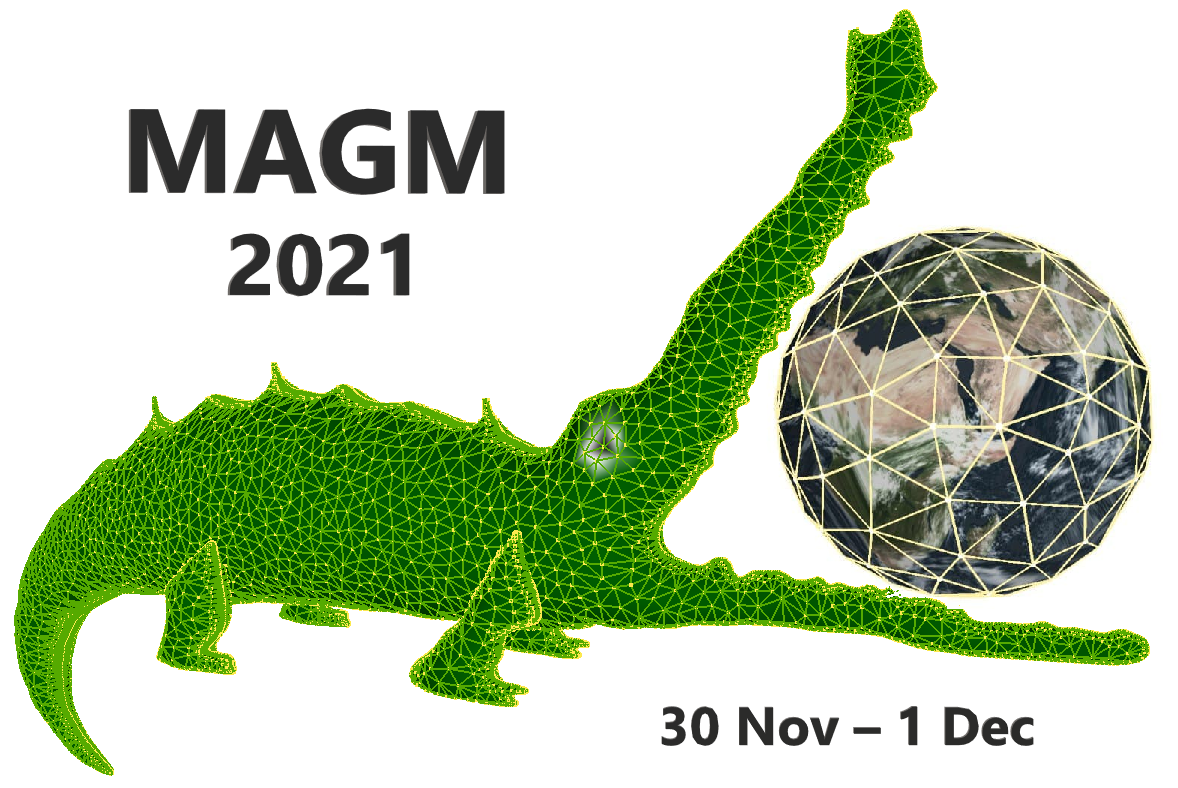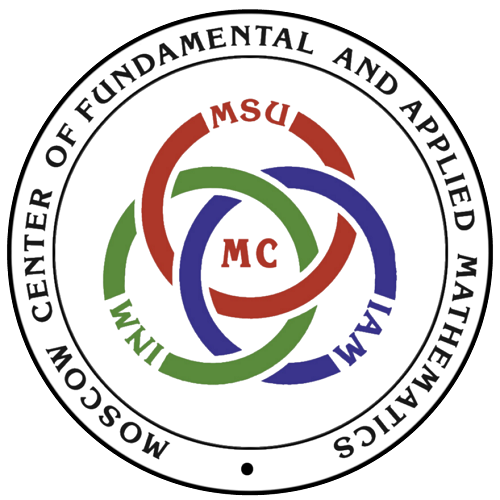﻿ Laboratory site
En / Ru

# International Workshop on Multivariate Approximation and Geometric Modeling

Workshop on Multivariate Approximation and Geometric Modeling (MAGM-2021) will be held on November 30 and December 1 online (via Zoom). All are invited to attend.The workshop is organized in cooperation with Moscow Center of Fundamental and Applied Mathematics.

Registration form (to get Zoom link)

Program (Moscow time, UTC+3):

Tuesday, November 30

→ 17:00-18:00 Harmut Prautzch, "On cutting convex polyhedra";

Abstract In Chaikin's algorithm, we iteratively smooth a polygonal line by cutting corners such that we arrive at a quadratic $$C^1$$ spline in the limit. For this and other edge preserving corner cutting schemes, we have de Boor's most general result that the limiting curve is $$C^1$$ if and only if the maximum angle flattens out eventually. In this talk, I will discuss face preserving cutting for convex polyhedra, explain why de Boor's result can not easily be generalized, go through counter examples and present results. Further, I introduce our 4-8 and 4-6-8 schemes, show outcomes of ongoing experiments, and may also briefly mention our honeycomb and $$\sqrt{3}$$ cutting schemes.

→ 18:00-19:00 Elisabeth Larsson, "Simulating the human respiratory system from an approximation perspective";

Abstract When intensive care patients are put in a ventilator, both the lungs and the diaphragm, which is the main respiratory muscle, are damaged by the mechanical ventilation. This prolongs the intensive care period and is an undesired side effect. To understand these effects better, we want to simulate both natural and mechanical ventilation. This is a hard problem and here we describe the steps that we have made so far. We have chosen to use radial basis function (RBF) methods to build our model. The reason is that the methods are flexible with respect to geometry, they can be computationally efficient due to the potential high-order accuracy, and they are easy to use also for complex problems. We discuss two aspects, first building a smooth geometry from medical image data, and then RBF simulation methods that are adapted to thin geometries. We present theoretical convergence results and numerical experiments that confirm the theoretical findings.

→ 19:00-20:00 Thomas Mejstrik, "Multiple subdivision schemes and joint spectral radius".

Abstract Subdivision schemes are a method to generate smooth curves and surfaces by iteratively refining a mesh of points which eventually converge to a continuous limit.
For multiple subdivision schemes, in every refinement step different rules for data and mesh generation are allowed. New data is produced by convolution of old data with uniformly bounded sequences, called masks, the underlying mesh is modelled as a lattice which gets refined by expanding matrices, called dilation matrices.
We show how the joint spectral radius of a finite set of square matrices, derived from the refinement masks and dilation matrices, characterises the convergence properties of the underlying multiple subdivision scheme.
Last we discuss the invariant polytope algorithm, and modifications thereof, which can be used to compute the joint spectral radius in reasonable time.Wednesday, December 1

→ 17:00-18:00 Ulrich Reif, "Interpolation and Approximation with Multivariate Polynomials and Splines";

Abstract In the first part of the talk we discuss error estimates for polynomial interpolation on tensor product grids. Unlike in the univariate case, constants can grow unboundedly when groups of break points exceeding a certain size collapse towards single points.
In the second part, we present Bramble-Hilbert type estimates in anisotropic Sobolev spaces concerning polynomial approximation of functions defined on domains. We extend known results by specifying explicit constants for domains bounded by diffeomorphic images of graphs.
Finally, we discuss some complications arising for the approximation of functions defined on domains by tensor product splines. In the bivariate case, so-called diversified B-splines provide a solution, but a counterexample shows that some problems are persistent in higher dimensions.

→ 18:00-19:00 Costanza Conti, "Perspectives and open problems for non-stationary subdivisions";

Abstract Subdivision schemes were created originally to design geometrical models but very soon they were recognized as methods for approximation. They are iterative methods for points generation based on refinement rules that can be easily and efficiently implemented on a computer. Since the 90s, subdivision schemes attracted many scientists for both the simplicity of their basic ideas and the mathematical elegance emerging in their analysis: they are defined by repeatedly applying simple and local refinement rules which have been extended to refine other objects such as vectors, matrices, sets of points, curves, nets of functions, or manifold data. Therefore, the domain of application of subdivision is vast and they emerge in different contexts ranging from computer animation to motion analysis.
The most studied subdivision schemes are linear and stationary (level independent). A nice aspect of linear subdivision schemes is that many of their properties can be translated into algebraic properties of Laurent polynomials. This makes the verification of some of their properties easy.
Moreover, since these schemes can be viewed as repeated multiplication by matrices, many analysis tools are based on linear algebra such as the "joint spectral radius" of two matrices. Stationary schemes are characterized by repeatedly applying the same simple and local refinement rule while the non-stationary (level dependent) schemes apply a different rule in each level of refinement. But, the non-stationary schemes are equally simple to implement and highly intuitive in use: from an implementation point of view changing rules with the levels is not a crucial matter, also in consideration of the fact that, in practice, only a few subdivision iterations are performed.
Non-stationary subdivision schemes were originally introduced with the aim of enriching the class of limit functions of stationary schemes. For example, in the univariate case, they can generate exponential B-splines or $$C^\infty$$-compactly supported functions like the Rvachev function. Actually, these schemes have very different and distinguished properties: while stationary subdivision schemes are not capable of generating conic sections, or dealing with level-dependent tension parameters to modify the shape of a subdivision limit, non-stationary schemes are able to. Moreover, level-dependent subdivision schemes include Hermite schemes that do not model only curves and surfaces, but also their gradient fields and are considered of interest both in geometric modeling and biological imaging. Additionally, non-stationary subdivision schemes are at the base of the construction of non-stationary wavelets and framelets that, being level adapted, are certainly more flexible. Last, but not least, level-dependent rules could be used to overcome the standard limitations of subdivision surfaces such as artifacts and low regularity at extraordinary vertices/faces.

→ 19:00-20:00 Carla Manni, "Tchebycheffian B-splines in Isogeometric Analysis".

Abstract Tchebycheffian splines are smooth piecewise functions with sections in spaces more general than classical algebraic polynomials. Interesting examples are spaces comprising trigonometric or hyperbolic functions. Under suitable assumptions, generalized splines enjoy all the desirable properties of polynomial splines, including a representation in terms of basis functions (the so-called TB-splines) that are a natural extension of the polynomial B-splines.
Isogeometric analysis (IgA) is a well-established paradigm for the analysis of problems governed by partial differential equations. It provides a design-through-analysis connection by exploiting a common representation model. This connection is achieved by using the functions adopted in Computer Aided Design (CAD) systems not only to describe the domain geometry, but also to represent the numerical solution of the differential problem. CAD software, used in industry for geometric modeling, typically describes physical domains by means of tensor-product B-splines and their rational extension, the so-called NURBS. In its original formulation IgA is based on the same set of functions. Nonetheless, the IgA paradigm is not confined to B-splines, NURBS and their localized extensions.
Thanks to their complete structural similarity with classical B-splines, TB-splines are plug-to-plug compatible with B-splines in IgA. On the other hand, when dealing with TB-splines, the section spaces can be selected according to a problem-oriented strategy taking into account the geometrical and/or analytical peculiar issues of the specific addressed problem. The fine-tuning of the approximation spaces results in a gain from the accuracy point of view.
In this talk we review some isogeometric methods based on Tchebycheffian splines of relevance in practical applications, discussing the differences and the similarities with the polynomial case.

## Lecturers:

Organizing commitee:

V.Yu. Protasov (chair)

V.N. Temlyakov (chair)

→ V.E. Podolskii

T.I. Zaitseva (scientific secretary)

Contacts: approx.lab.msu@gmail.com